# Catch up on the latest AI articles# TAPAS: A Language Model For Reasoning On Tabular Data

3 main points
✔️ Proposed a language model TAPAS for question answering on tabular data
✔️ Pre-trained on a large dataset of text-table pairs, fine-tuning on a semantic parsing dataset
✔️ outperformed existing methods on semantic parsing tasks performance over existing methods on a semantic parsing task.

written by Jonathan HerzigPaweł Krzysztof NowakThomas MüllerFrancesco PiccinnoJulian Martin Eisenschlos
(Submitted on 5 Apr 2020 (v1), last revised 21 Apr 2020 (this version, v2))

Subjects:  Information Retrieval (cs.IR); Artificial Intelligence (cs.AI); Computation and Language (cs.CL); Machine Learning (cs.LG)code：The images used in this article are from the paper, the introductory slides, or were created based on them.

## Introduction

The problem of answering natural language questions about tabular data has been treated as a semantic parsing problem. (Semantic parsing is the process of replacing questions with logical operations.) ) The lack of annotated data has been an issue in supervised learning of this problem.

In this paper, we propose a weakly supervised learning model Table Parser (TAPAS) that can reason and answer questions on tabular data.

## Model implementation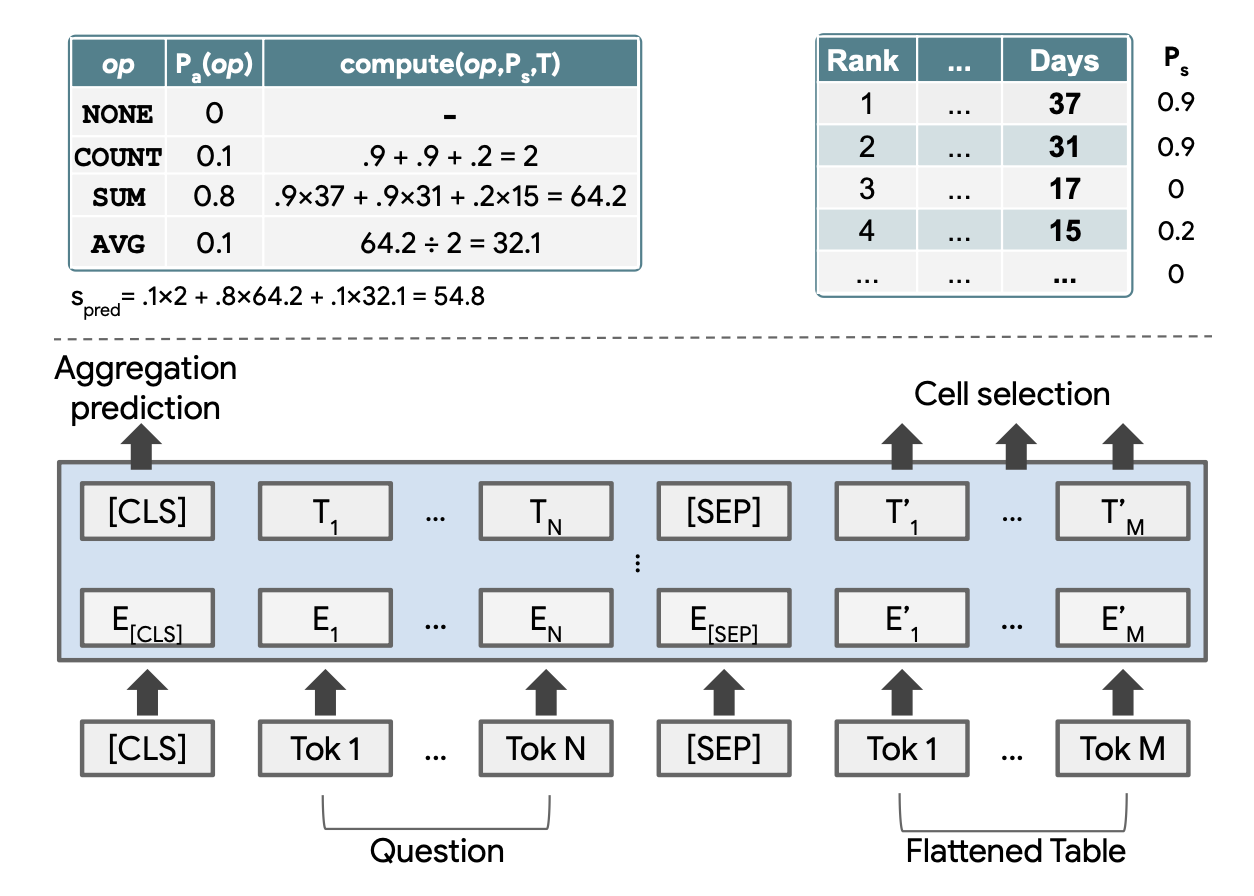The figure above is a schematic of the proposed model process.

To encode the table structure, the structure adds its own positional encoding to the BERT encoder model.

The tabular form is smoothed into a series of words and concatenated with the question text for input. For the output portion, an output layer is added to select the cells to be manipulated and to predict the arithmetic operations.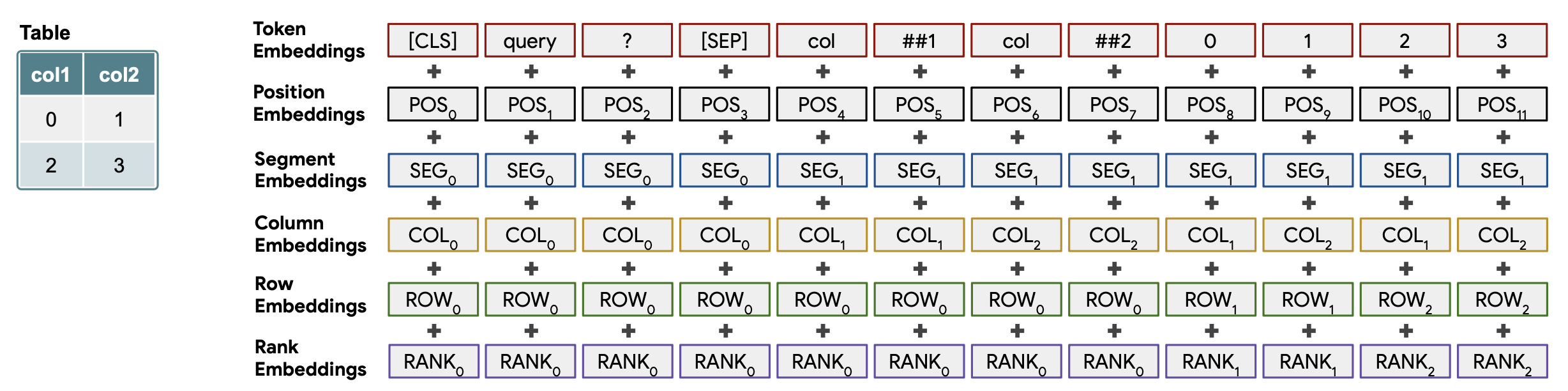As shown in the figure above, several embeddings are added to the series of word and table data (Token Embeddings).

Position Embeddings
Similar to BERT, these are embeddings of positions in a series.

Segment Embeddings
Embeddings to distinguish between queries and tabular data. The former corresponds to 0 and the latter to 1.

Column Embeddings / Row Embeddings
Embeddings are the indexes (starting from 1) of rows and columns in a table. For a query sentence, 0 corresponds to 0.

Rank Embeddings
If the column data in the table is a floating-point number or a date, it is given an ordinal rank in ascending order. Numbers that are not comparable are numbered with 0, and numbers are assigned in descending order from 1 to the smallest value. This is a countermeasure to the problem that it is difficult to directly compare numeric data as linguistic information.

This is an embedding used to refer to the previous question or answer when the question and answer are repeated in an interactive manner. The answer is assigned 1 and all others are assigned 0.

### On the output layer for cell selection

operator, which outputs the cell to be operated on.

Each cell is modeled as an independent Bernoulli variable and outputs the probability that the cell is selected. Cells with probability greater than 0.5 are selected.

### For the output layer to predict the arithmetic operations

Outputs operations such as SUM, COUNT, AVERAGE, and NONE.

## Prior learning

The TAPAS model is pre-trained using large tabular data sets from Wikipedia.
To do this, we create a dataset by extracting text and tabular data pairs from Wikipedia.
Table captions, article titles, descriptions, etc. are used in place of questions.
This data is then used to train for the Masked language model task.

## Fine-tuning

Suppose that the training dataset consists of $N$ samples. The samples are pairs $(x, T, y)$ consisting of tabular data $T$, a question $x$ and its answer $y$.
The goal of the model is that given tabular data $T$ and a question $x$, it should be able to select the appropriate cell and perform the appropriate operation $z$ to produce the correct answer $y$.

In the following, we will discuss each task in detail. Suppose that the answer $y$ is written as a tuple of the coordinates $C$ of the cell to be selected and the correct scalar value $s$ ($y = (C, s)$).

### Cell selection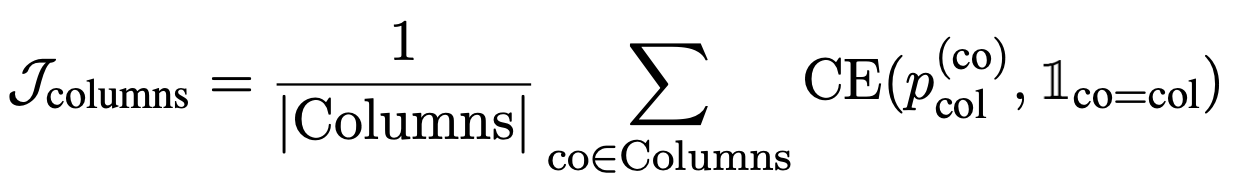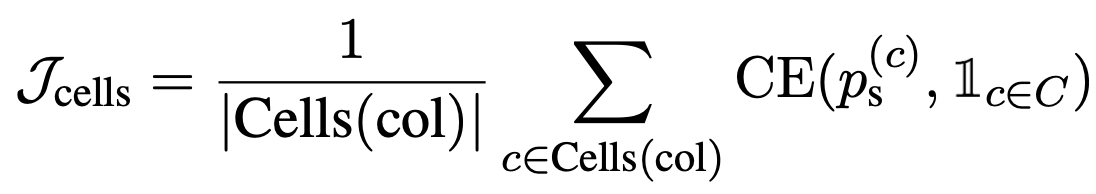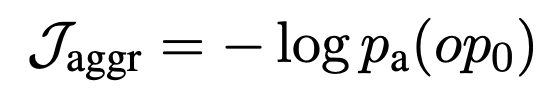Losses $\mathcal{J}_\mathrm{columns}$ and $\mathcal{J}_\mathrm{cells}$ that indicate whether the appropriate columns and appropriate cells have been selected, plus losses $\mathcal{J}_\mathrm{CS}$ that indicate whether the appropriate operation NONE has been selected. aggr}$, plus the loss$\mathcal{J}_\mathrm{CS} = \mathcal{J}_\mathrm{columns} + \mathcal{J}_\mathrm{cells} + \alpha \mathcal{J}_\mathrm{aggr} and perform the optimization.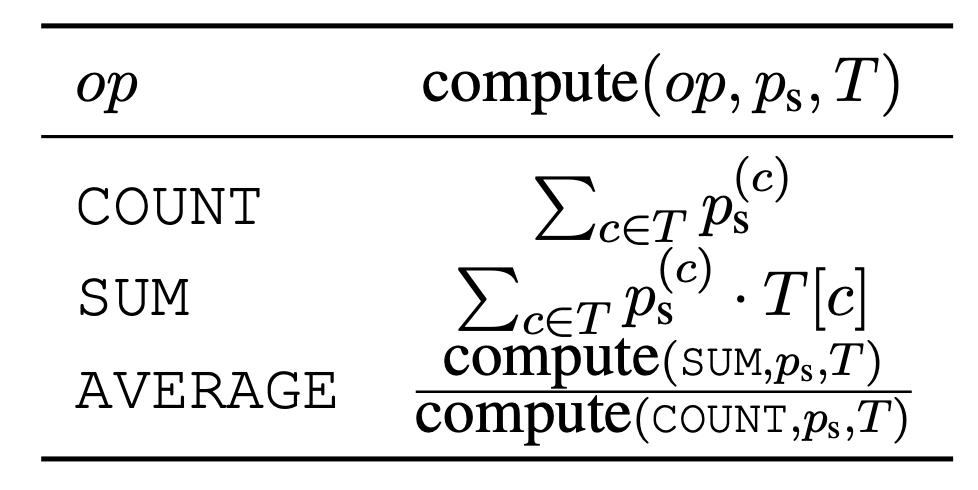As shown in the table above, the various operations (COUNT, SUM, AVERAGE) are expressed in differentiable form.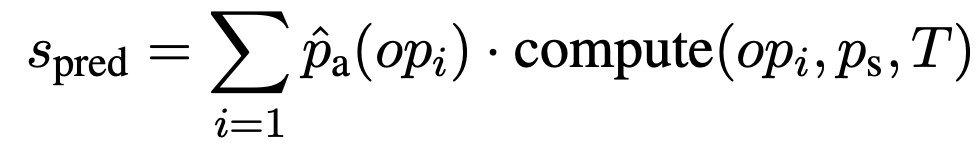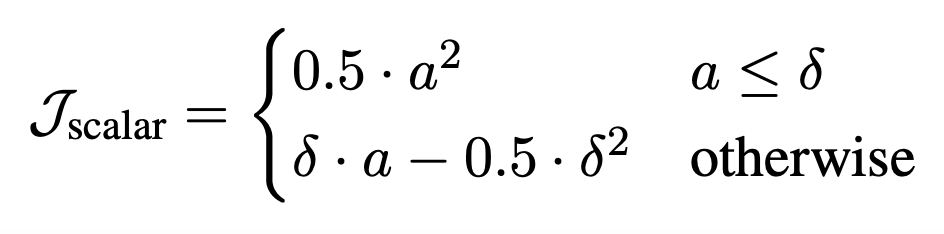Then, the absolute value of the difference between the result $S_{\mathrm{pred}}$ and the correct scalar value $S$ of the selected operation applied to the selected cell is calculated as $A$, and the loss $\mathcal{J}_\mathrm{scalar}$ is calculated to indicate whether the resulting scalar value is appropriate.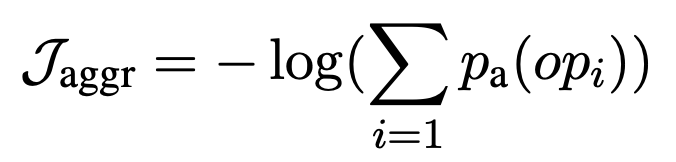In addition, we compute the loss $\mathcal{J}_\mathrm{aggr}$, which indicates whether the appropriate operation is selected, and perform optimization using the loss $\mathcal{J}_\mathrm{SA} = \mathcal{J}_\mathrm{aggr} + \beta \mathcal{J}_\mathrm{scalar}$, which is the sum of the two losses.

## Experiment

### Data-set

We experimented with several datasets for semantic parsing, including the following three

WIKITQ is a dataset of table data from Wikipedia with various questions and answers.

SQA is a dataset that breaks down the WIKITQ questions and replaces them with simple questions.

WIKISQL is a dataset that contains operations and questions for SQL.

### Experimental setup

We use a lexical tokenizer (standard BERT tokenizer) consisting of 32,000 words; we started pre-training and fine-tuning with the BERT-Large model.

### Experimental results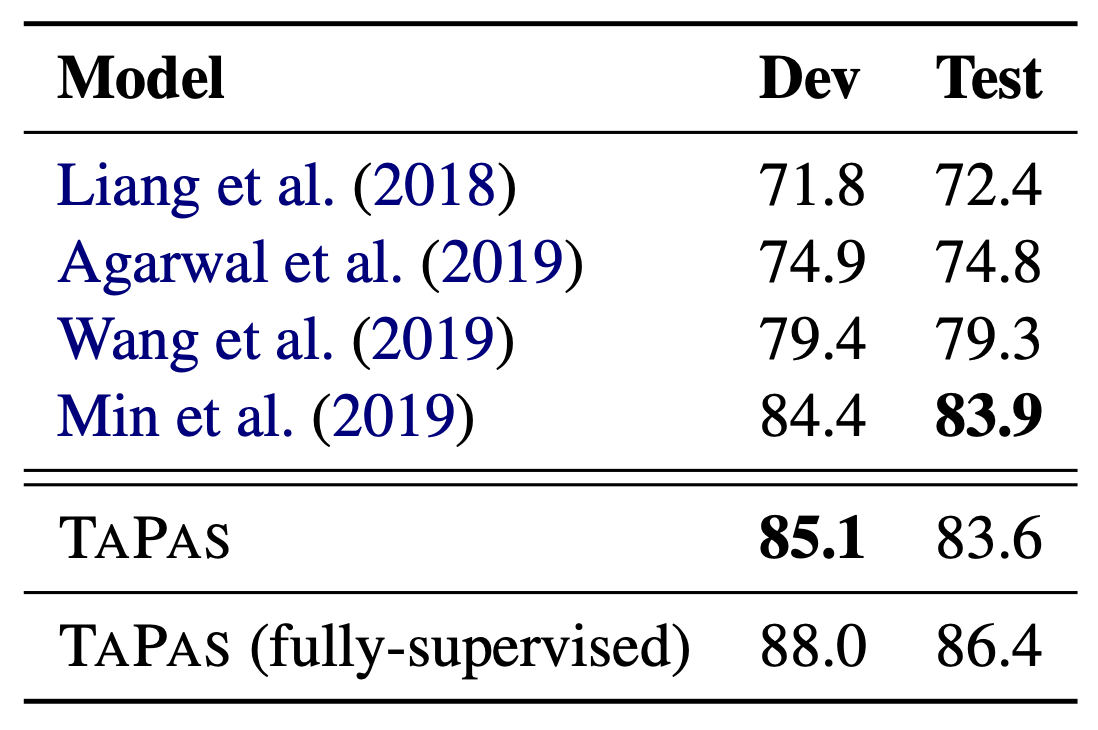The table above shows the performance against WIKISQL, indicating that TAPAS performs as well as state-of-the-art methods.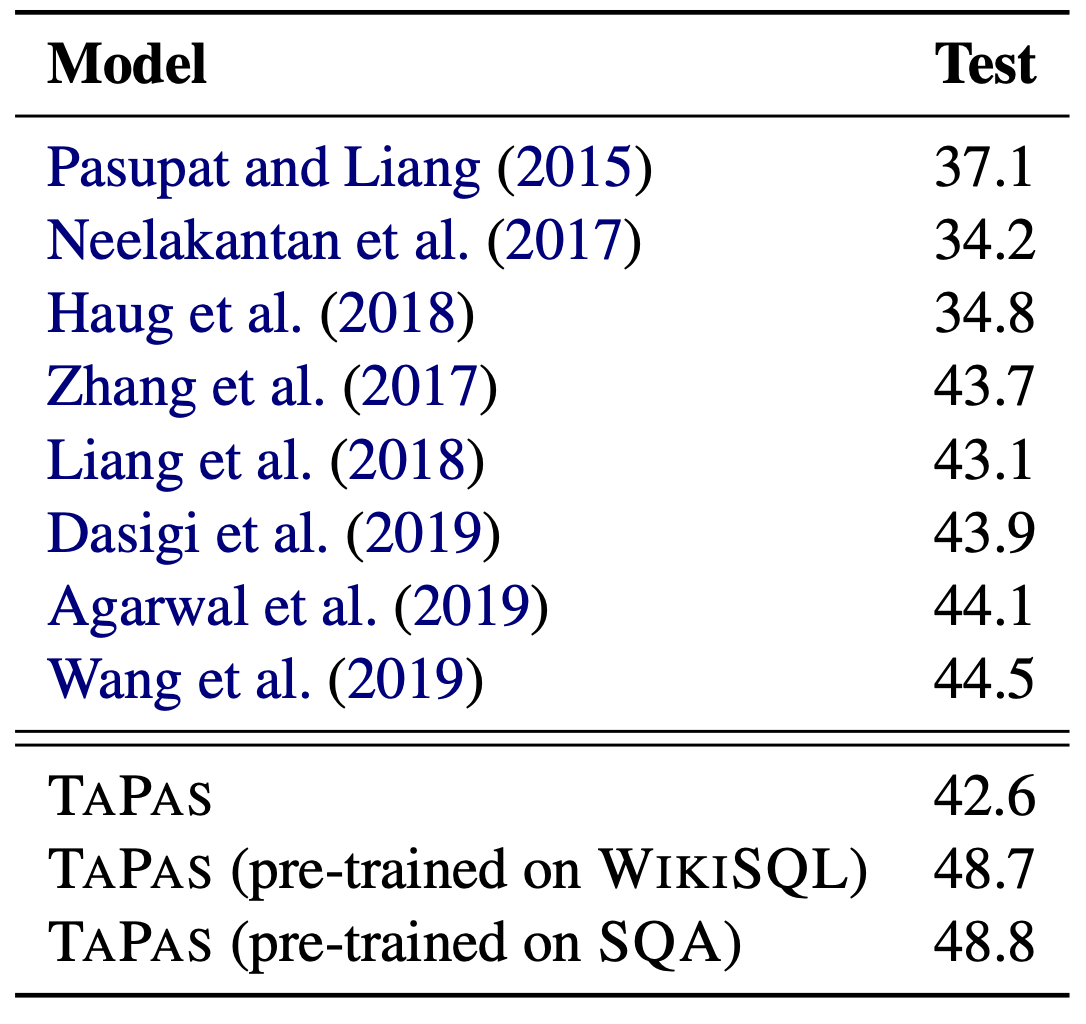The table above shows the performance against WIKITQ, with TAPAS performing best when pre-trained with WIKISQL or SQA.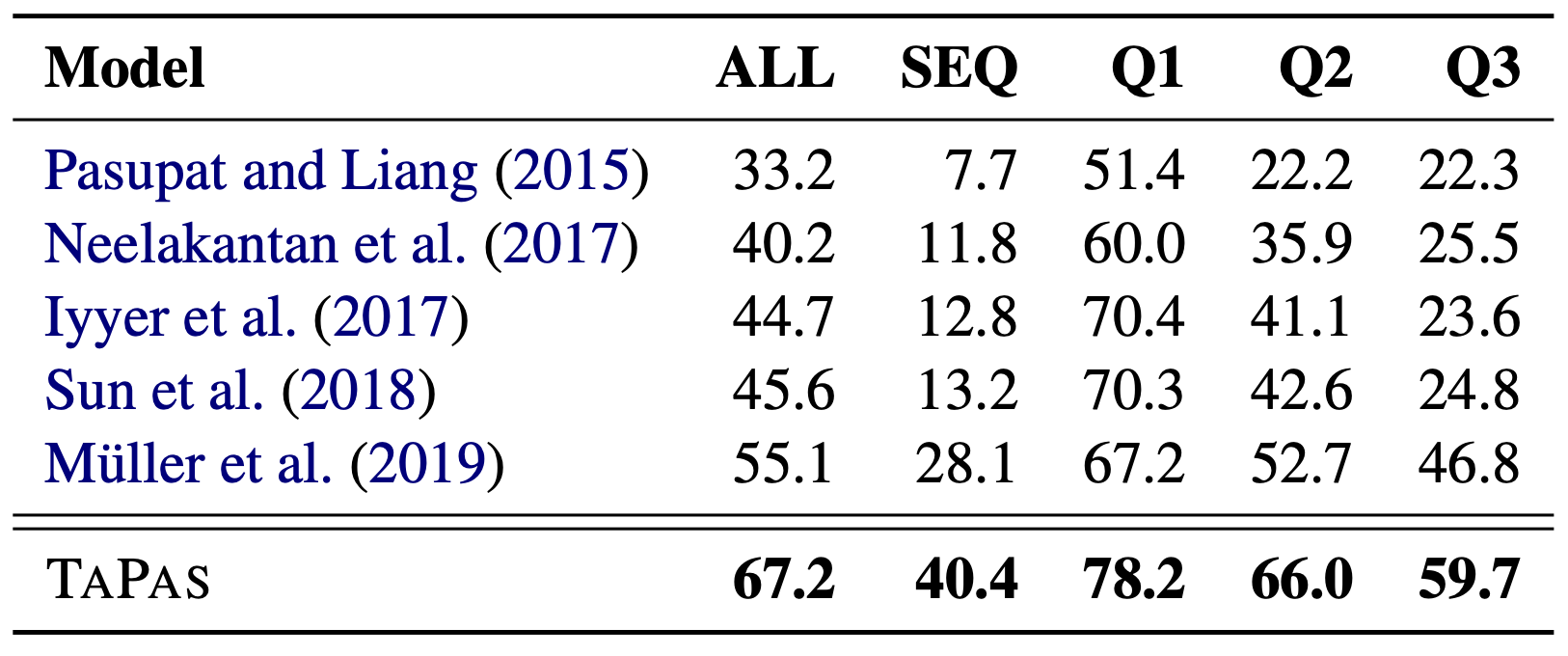The table above shows the performance against SQA, with TAPAS performing the best on all indicators.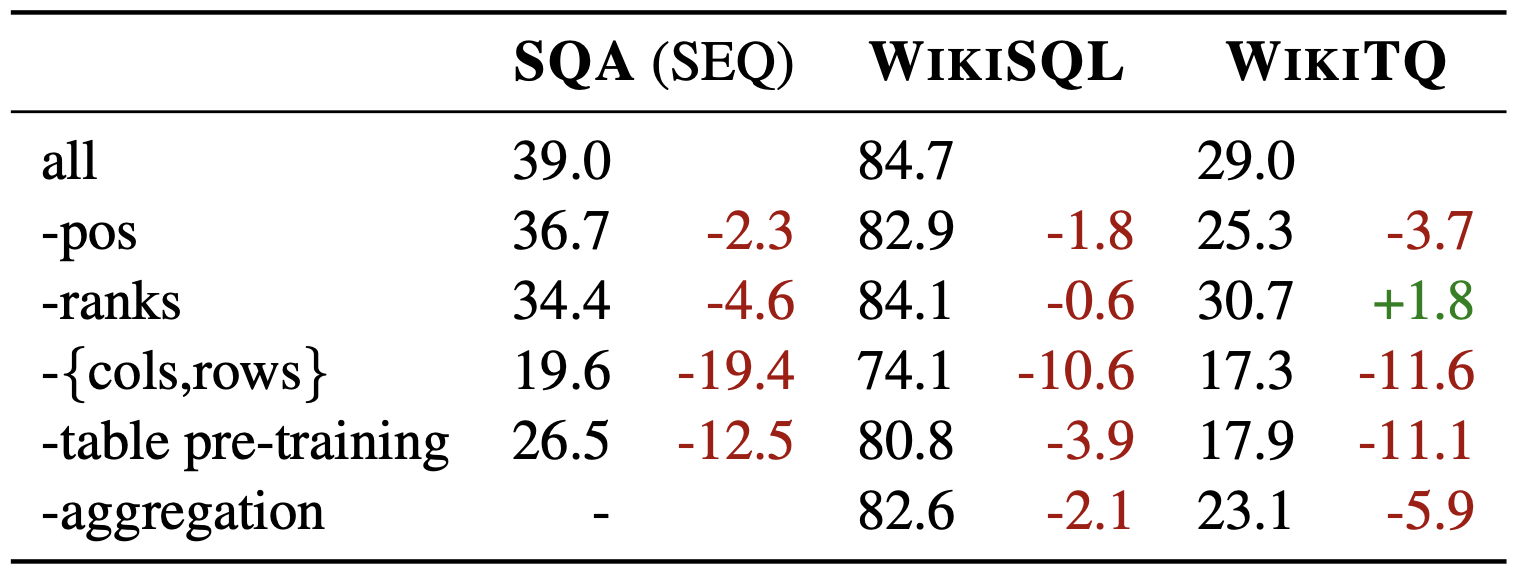The table above shows the results of an ablation study that shows how performance declines when each embedding, pre-training, etc. is omitted.

It can be read that pre-training on tabular datasets and position embedding of columns and rows were important to improve performance.

## Limit

The method proposed in this paper is not applicable to data containing multiple tables due to the limited size of tabular data that can be handled, since the model is fed a single tabular data as a string. Also, due to its formulation, it cannot handle complex processes that require more than two steps, such as comparisons of averages.

## Summary

The model TAPAS introduced here is a BERT-based model that proposes an effective method for embedding tabular data, although task-specific fine-tuning is required. Further development in this field is expected in the future.

If you have any suggestions for improvement of the content of the article,